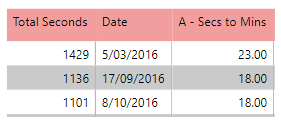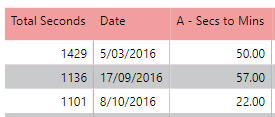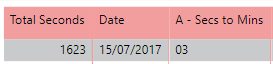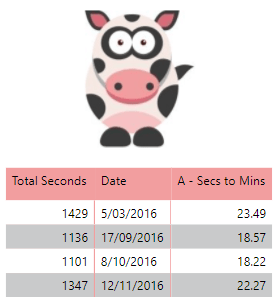I was recently doing some consulting work for a customer and they had a column which contained seconds and whilst this is useful it is better to have it shown as Minutes and Seconds, which is easier to relate and compare.

Below I will show you how I came up with the DAX measure which converts it from seconds to minutes, and I will be using my Parkrun sample dataset. As shown below I have a table which shows the total secondsBelow is my DAX measure with an explanation on how I got to the measure

```Secs to Mins =
VAR TimeSecs =
SUM ( 'ParkRun'[Total Seconds] )+1
VAR TimeMins =
DIVIDE ( TimeSecs, 60 )
VAR JustSeconds =
TimeMins - TRUNC ( TimeMins )
VAR JustMinutes =
TimeMins - JustSeconds
VAR JustSecondsInTime = JustSeconds * 60
VAR MySeconds2 =
IF ( JustSecondsInTime < 10, FORMAT ( "0" & JustSecondsInTime, "00" ), JustSecondsInTime )
RETURN VALUE ( JustMinutes  & "." & LEFT ( MySeconds2, 2 ) )```
• Line 1
• This is where I created the measure with a name
• Lines 2 – 3
• This is where I am summing up all the Total Seconds
• Lines 4 – 5
• I am creating a variable called TimeMins and then dividing the seconds by 60 to get the minutes.
• Line 6 – 7
• I am creating a variable called JustSeconds, and I am using the TRUN DAX function, so that this returns the remainder of the variable TimeMins, which is currently stored as a decimal.
• NOTE: The above means that the remainder is stored as decimal point out of 100. So if the remainder is 0.50 that translates to 30 seconds. Which is shown below.
•• Line 8 – 9
• I am creating a variable called JustMinutes, where all that I want is the Minutes, so I do this by subtracting the TimeMins from JustSeconds
•• Line 10
• I am creating a variable called JustSecondsInTime, and what I am doing is converting the seconds from a decimal value back to time.
• What this means is that if the decimal value was 0.50, the variable JustSecondsInTime will be converted to 0.30 which represents 30 seconds.
•• Line 11 – 12
• I am creating a variable called MySeconds2, and what I am doing here is to ensure that if it is less than 10 seconds to add in a zero before the second number. This is so that it will be displayed as expected.
• As shown below you can see where the seconds are less than 10 it is displaying correctly.
•• When looking at the image above it does appear that the seconds is not being displayed correctly. This will be resolved in the next step below.
• Line 13 – 14
• Now I am closing off the variable section to return the measure.
• I begin with the DAX function called VALUE, this is because I want my DAX to be a measure in my model.
• I then put in my variable called JustMinutes which is the minutes from the seconds that I defined in lines 8 & 9
• Next, I put in a dot “.” This is to ensure that the VALUE will still work because it expects a value in the format of 99.22
• If I had to change it from a dot to the colon the VALUE function would return an error.
• I get the seconds to display correctly by using the DAX function LEFT ( MySeconds2, 2 )
• What I am doing here is using the LEFT DAX Function to get the first 2 values starting from the left hand side. Which results in displaying the seconds
•Now when I take my measure and put it into a table I get the Minutes and seconds displaying as expected.I found that I really had to use the DAX variables to get this pattern to work as expected. With all the screenshots above, I changed the return function to the particular DAX variable that I was working on to ensure that it was returning the value that I was expecting.

If you have any questions or comments please leave them in the section below. I do hope that you found this useful.